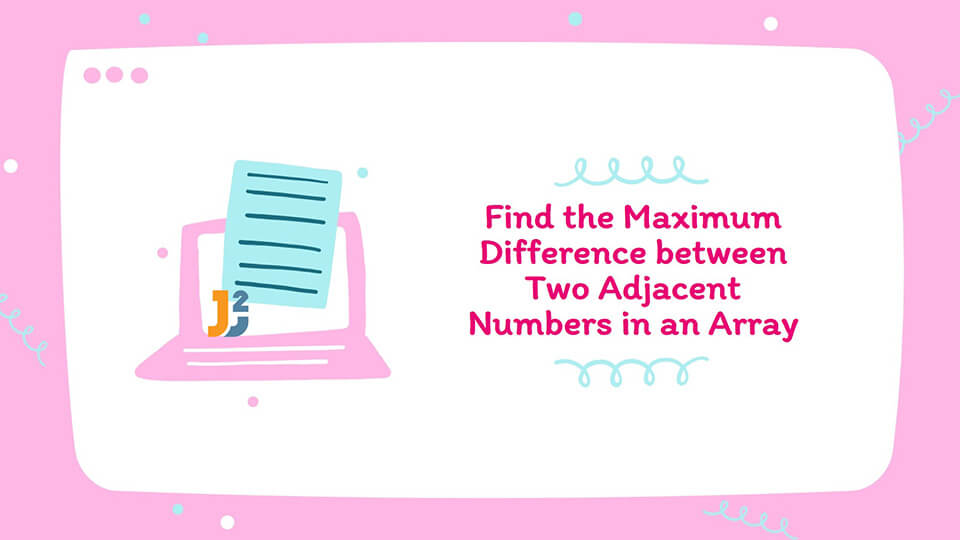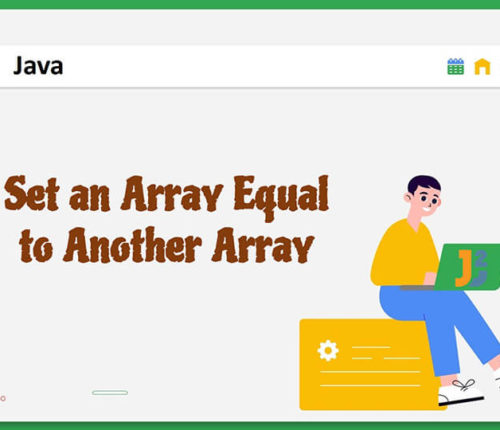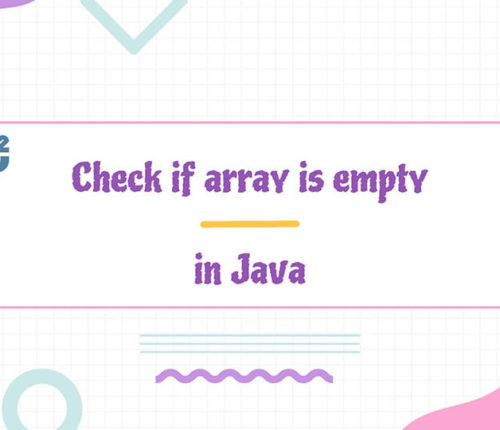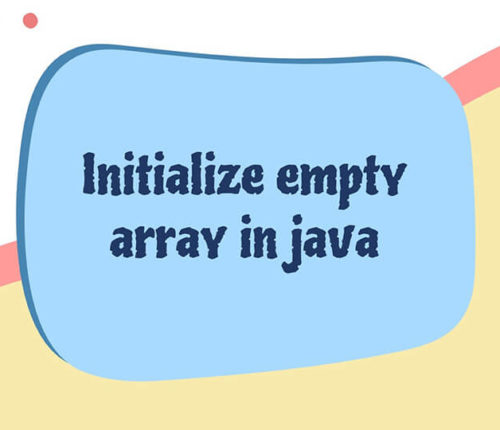# Write a Program to Find the Maximum Difference between Two Adjacent Numbers in an Array of Positive IntegersIn this article, we look at a problem : `Given an Array of Positive Integers, Find the [Maximum Difference](https://java2blog.com/maximum-difference-between-two-elements-in-array/ "Maximum Difference") between Two Adjacent Numbers`.

For each pair of elements we need to compute their difference and find the Maximum value of all the differences in array.

Let us look at an example, Consider this array:

For the above shown array, we have to solve the given problem. Here are the steps which we will follow for the implementation:

• Firstly, for each pair of elements, we have calculated their difference. We have calculated the Absolute Value of their difference because the array is not sorted and elements on either side can be greater or smaller.
• In the figure above, we have shown the Diff. value for each such pair. We need to find the Maximum Value among these which for the above case is : `11` for the pair `(1,12)`.
• We are going to iterate through the array, Arr starting from index 1 and calculate the current difference for each pair as : `Arr[i]>Arr[i] - Arr[i-1]`code>. We have a `max_diff` variable which maintains the maximum of all the differences, on each iteration we update the `max_diff` with current difference.

`Note:` To calculate the Absolute Value we use `Math.abs()` in java. The approach is for only Positive Values in the array.

Now, let us have a quick look at the implementation:

`Output:`

We implement the same example discussed above in code. Let us analyze the complexity of the code.

`Time Complexity:` We do a simple single traversal of the array which takes linear time so the time complexitiy is `O(n)`, for n elements in array.

That’s it for the article you can try out this code with different examples and let us know your suggestions or queries.

## Related Posts

•23 April

### Set an Array Equal to Another Array in Java

Table of ContentsSetting an Array Variable Equal to Another Array VariableSet an Array Equal to Another Array in Java Using the clone() MethodSet an Array Equal to Another Array in Java Using the arraycopy() MethodSet an Array Equal to Another Array in Java Using the copyOf() MethodSet an Array Equal to Another Array in Java […]

•04 March

### Check if Array Is Empty in Java

Table of ContentsCheck if the Array Is Empty in JavaThe Array Variable Has the Null ReferenceThe Array Does Not Contain Any ElementThe Array Has Only Null ElementsUsing the Java Library to Check if the Array Is Empty in JavaUsing Apache Commons Library to Check if the Array Is Empty in JavaConclusion In this post, we […]

•01 January

### Initialize empty array in java

Table of ContentsIntroductionHow do you initialize an empty array in java?Using new Keyword with predefined Values and SizeUsing Anonymous Array Objects to initialize empty arrayUsing java.util.Scanner Class for user input with predefined size. Using java.io.BufferedReader to initialize array for user input with unknown sizeUsing fill() method of java.util.Arrays Class to initialize empty array 💡 Outline You […]

•11 November

### How to print array in java

Learn about how to print array in java in multiple ways.

•09 October

### Initialize 2D array in Java

Table of ContentsInitialize 2D array Using for loopInitialize 2D array using an initializerInitialize 2D array of variable columns lengthInitialize 2D array with heterogeneous dataInitialize 2D array using initialilzer with columnsInitialize 2D array using Reflection APIInitialize 2D array using the toCharArray() In this article, we will learn to initialize 2D array in Java. The array is […]

•24 October